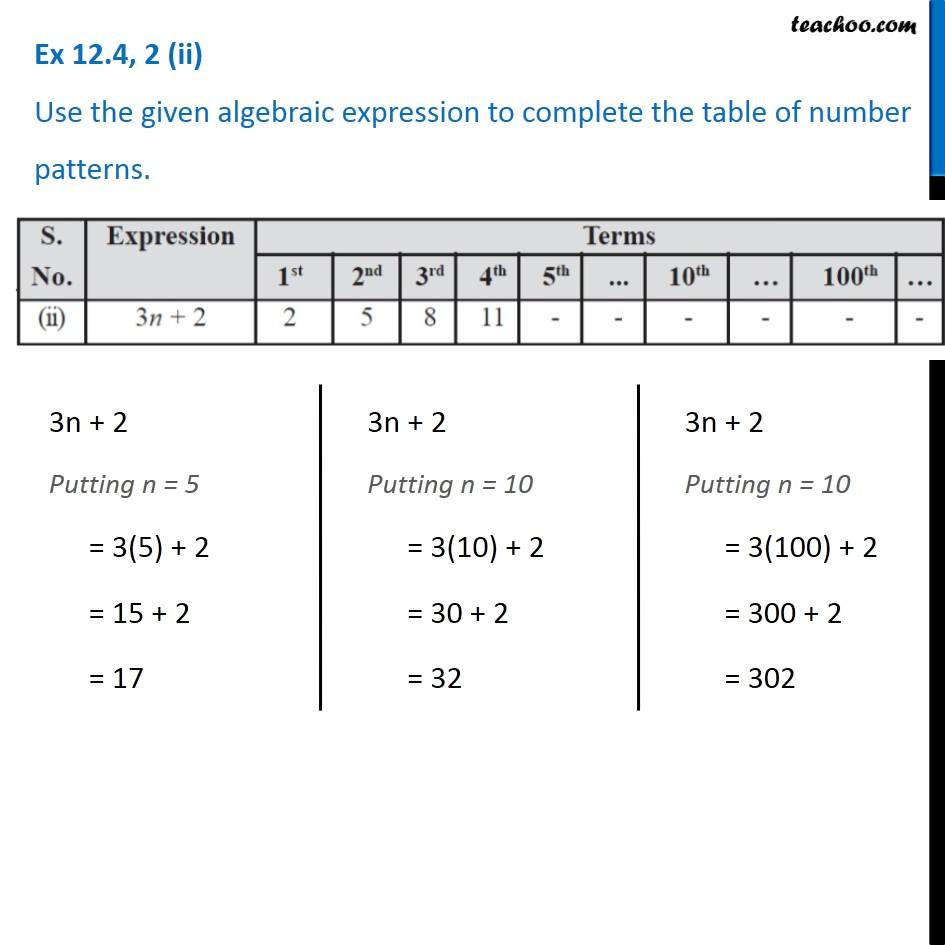Observing patterns

Chapter 10 Class 7 Algebraic Expressions
Serial order wiseLearn in your speed, with individual attention - Teachoo Maths 1-on-1 Class

### Transcript

Question 2 (ii) Use the given algebraic expression to complete the table of number patterns. 3n + 2 Putting n = 5 = 3(5) + 2 = 15 + 2 = 17 3n + 2 Putting n = 10 = 3(10) + 2 = 30 + 2 = 32 3n + 2 Putting n = 10 = 3(100) + 2 = 300 + 2 = 302